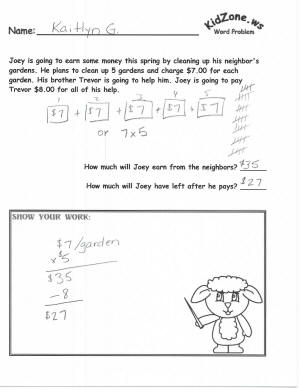# KidZone Math Spring Themed Word Problems

Contributed by Leanne GuentherThe spring word problem worksheets are listed by grade.

All of the spring word problems are dynamic (in other words, they regenerate a new problem each time you open them or click refresh on your browser).  The words in the particular problem will not change but the numbers will.

Children who struggle converting a word problem into a math equation will find it reassuring (confidence builder) to revisit the same verbal clues with different numbers.

When reviewing the spring word problems, you may find the math itself to be a little bit easier than you would expect from the grade.  This is intentional - keep in mind how challenging it is for the children to sift through the verbal clues and figure out what they need to do to the numbers provided to find the answers.

Please close the worksheet window when you have finished printing the worksheet.

## Grade 1 Spring Themed Word Problems:

1. Problem 1:  Addition of two numbers (between 0 and 10)
2. Problem 2:  Subtraction of two numbers (between 0 and 10)

## Grade 2 Spring Themed Word Problems

1. Problem 1:  Addition of two numbers (one between 0 and 99)
2. Problem 2:  Subtraction of two numbers (one between 0 and 99)

## Grade 3 Spring Themed Word Problems

1. Problem 1:  Multiplying single digit \$ amount plus addition of \$ without pennies
2. Problem 2:  Multiplying single digit \$ amount plus subtraction of \$ without pennies

## Grade 4 Spring Themed Word Problems

1. Problem 1:  Addition of three dollar amounts with pennies.
2. Problem 2:  Division by 2 (the fraction 1/2)

## Grade 5 Spring Themed Word Problems

1. Problem 1:  Long multiplication and simple division (answers have no remainders)
2. Problem 2:  Long multiplication and percentages (answers are whole numbers)